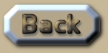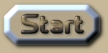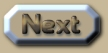Software Projects for Programmers

 Software Projects for Computer Students: 1) A simple algorithm with one body in orbit around another in real-time. 2) A binary pair of stars in orbit around each other. 3) Three or more solar bodies interacting with three or more gravity fields. (This requires a solution to the n-body problem for Newtonian gravity). 4) A solution to the problem of rotation curves of galaxies. 5) A Sun, Earth, and moon system. Such that the effect of the Sun’s gravity on the moon should be realized as a pull away from the Earth. This should not be to a correct scale in order to be more easily observed. 6) How the Solar System could have formed due to interaction between bodies of various sizes. Use random starting positions here. 7) How the Solar System probably formed due to one of a binary pair of stars going nova. The orbital velocity of the nova star gives rise to the orbital structure of the planets as it breaks apart. 8) How would the orbits of planets look around a binary pair of stars? 9) Examine how quantum time changes an eccentric orbit at perihelion. Is this quantum gravity? 10) Gravitational lensing. Demonstrate how the direction of parallel photons is changed by gravity such that gravity magnifies an image. 11) Examine the claims of the LIGO binary-black-hole merger in an evolutionary algorithm. This should have a scale with a 10% error-margin. 12) 12) How does General Relativity and Special Relativity change the shape of the orbits of the planets in the solar system? Also show how gravity moving at the velocity of light from General Relativity changes the orbital structure of a binary star system like Alpha Centauri. This requires sliding scales for distance and time with accuracy better than one part in a million-million. 13) An evolutionary algorithm of the solar system with an accurate sliding scale for time and distance which demonstrates an n-body solution to Newtonian gravity which is accurate to better than one part in a million. The precise pull by the Sun on the Moon’s orbit should be demonstrated, as well as how Jupiter changes the orbits of Mars and Mercury. 14) Binary Star formation. 15) Planetary ring formation. 16) Gee 17) Trojan asteroids. 18) Lunar orbital eccentricity. Is it a real or an algorithmic phenomenon? 19) Demonstrate aspects of the Big Bang in an algorithm showing how galaxies attain structure amidst the chaos of an explosion (or not chaos). 20) Simulate the movements of galaxies, and how they interact with one another. 21) Do it all from the start in 3d-space or even… 22) 4d-space. The first 12 steps I have completed. How far are you Mr Critic? .This is an extract summary of Chapter XXX of the book: Flight Light and Spin